# 08 学生课程分数的Spark SQL分析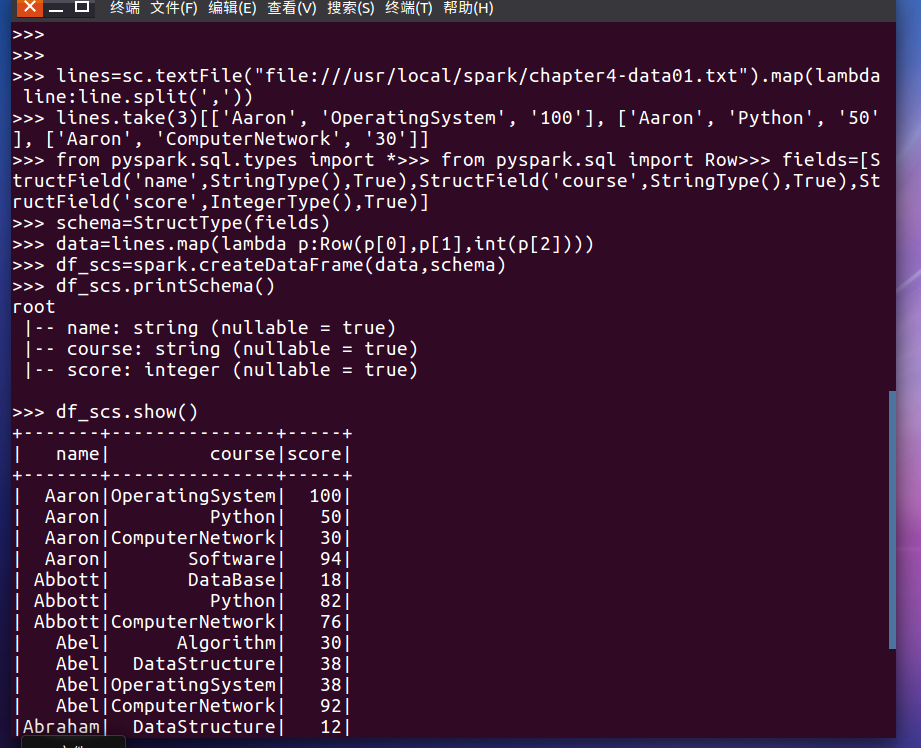• 每个分数+5分。
•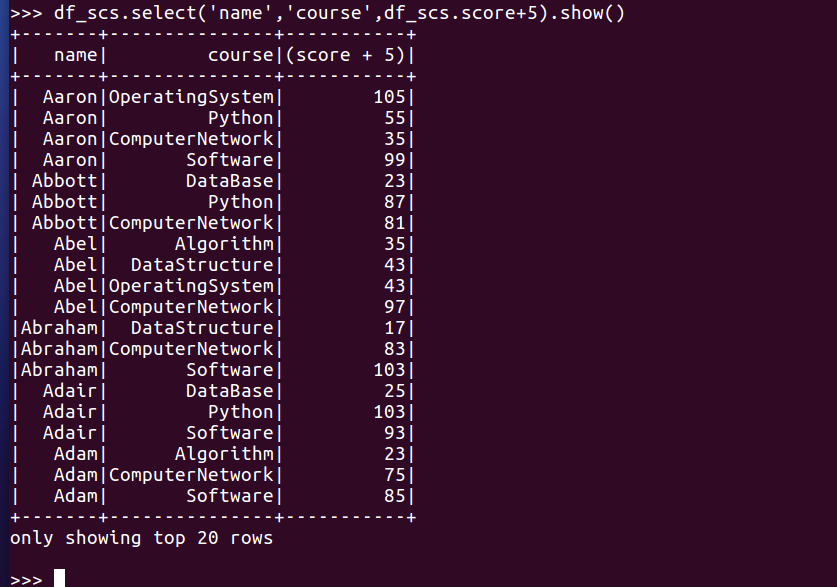• 总共有多少学生？
•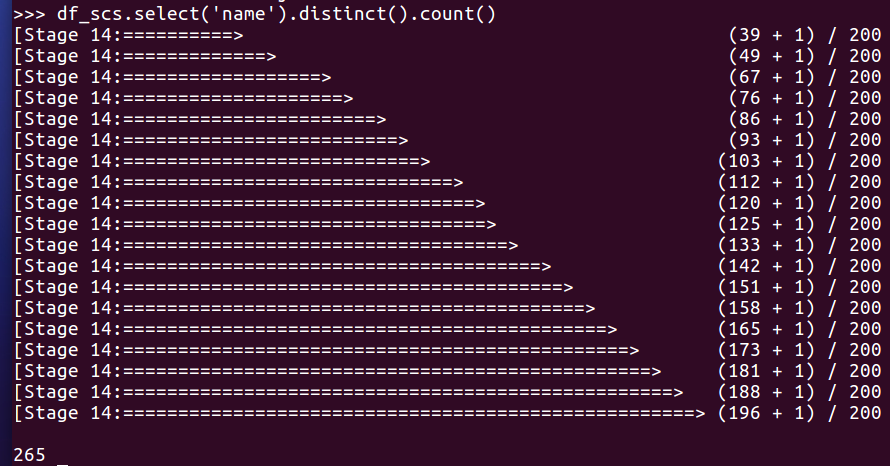• 总共开设了哪些课程？
•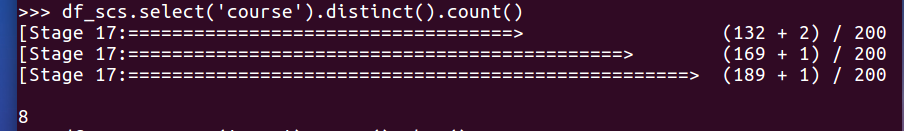• 每个学生选修了多少门课？
•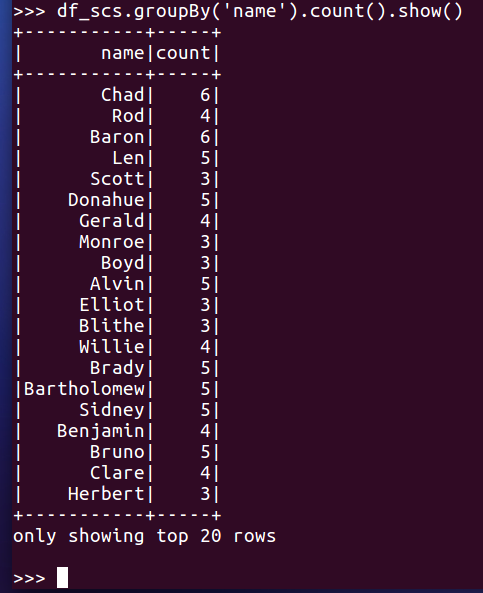• 每门课程有多少个学生选？
•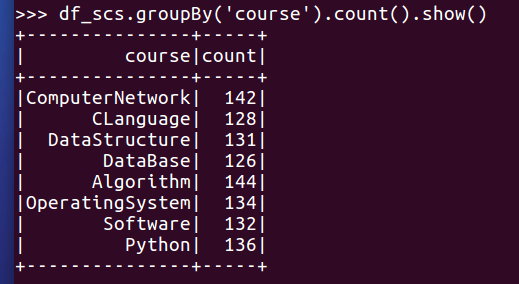• 每门课程大于95分的学生人数？
•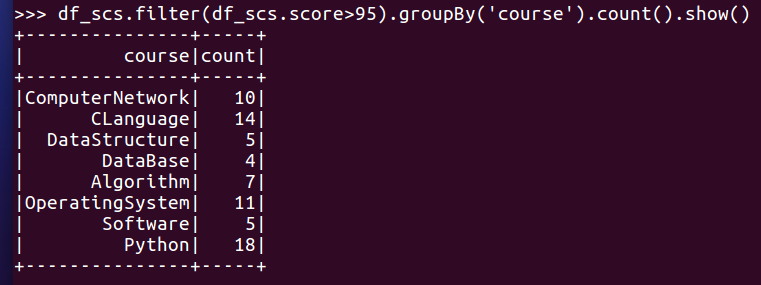• Tom选修了几门课？每门课多少分？
•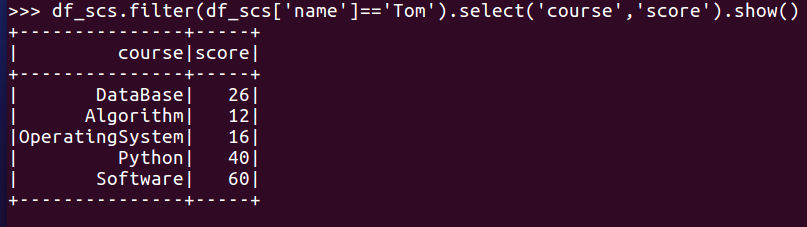• Tom的成绩按分数大小排序。
•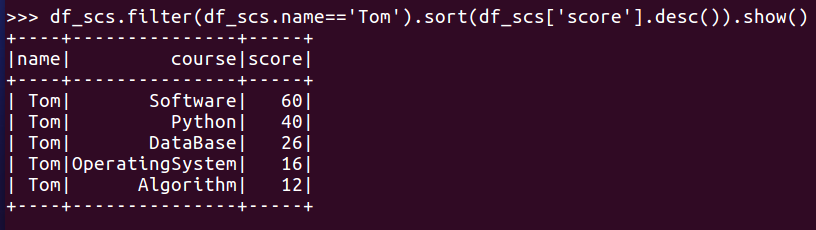• Tom的平均分。
•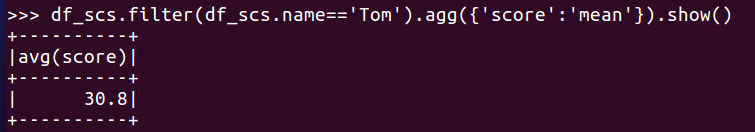• 求每门课的平均分，最高分，最低分。
•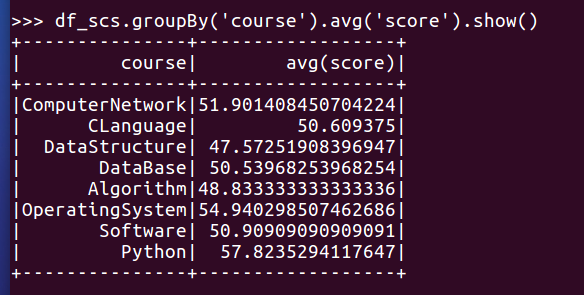•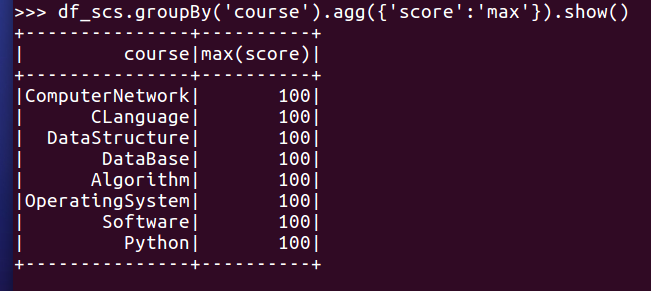•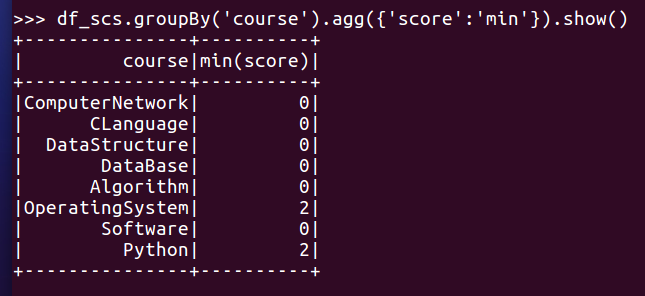• 求每门课的选修人数及平均分，精确到2位小数。
•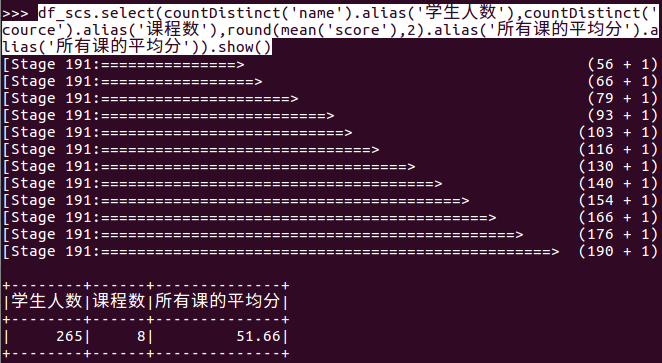• 每门课的不及格人数，通过率
•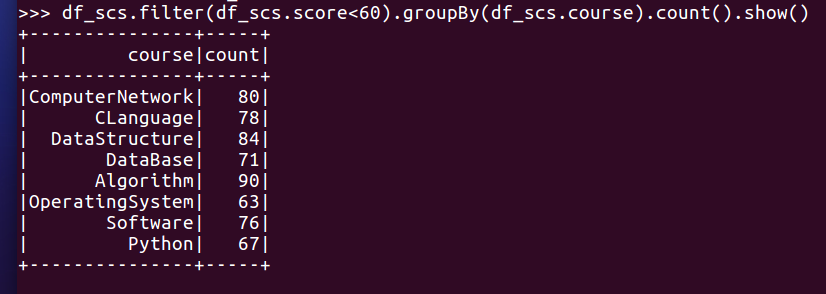• 结果可视化。

from pyspark.sql.types import IntegerType, StringType, StructField, StructType

fields = [StructField(...), ...]

schema = StructType(fields)

from pyspark.sql import Row

data = rdd.map(lambda p: Row(...))

Spark SQL DataFrame 操作

df.show()

df.printSchema()

df.count()

df.collect()

df[‘name’]

df.name

df.first().asDict()

df.describe().show()

df.distinct()

df.filter(df['age'] > 21).show()

df.groupBy("age").count().show()

df.select('name', df['age‘] + 1).show()

df_scs.groupBy("course").avg('score').show()

df_scs.agg({"score": "mean"}).show()

df_scs.groupBy("course").agg({"score": "mean"}).show()

posted @ 2021-05-20 18:55  不吃外卖  阅读(38)  评论(0编辑  收藏  举报